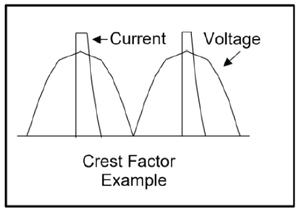# What is this Crest Factor Thing?

## Featured Product from Dataforth CorporationIn the early days of electrification, Edison distributed DC electric power. Tesla and Westinghouse had a better idea, AC. There was, and is, a need to understand the equivalence of the two. The most basic unit of interest is power measured in Watts. A Watt is a measure of the flow of energy in joules/second. The utility company billing unit is the kilowatthour, or 3,600,000 joules.

Wattage represents heating value. 100Volts DC into a 100Ohm resistor is 1Amp, and 100Volts x 1Amp = 100Watts. What AC value of voltage and current deliver 100Watts energy flow to the resistor? Call these Vrms and Irms, so 100Vrms x 1Arms = 100Watts. As they say in textbooks, “it can be shown” that the rms, or effective value called root mean square, of any periodic function is given by the following formula where T is the period.